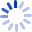# Infinite-Dimensional Manifolds: 1975 Lecture Notes

Robert Geroch's lecture notes "Infinite-Dimensional Manifolds" provide a concise, clear, and helpful introduction to a wide range of subjects, which are essential in mathematical and theoretical physics - Banach spaces, open mapping theorem, splitting, bounded linear mappings, derivatives, mean value theorem, manifolds, mappings of manifolds, scalar and vector fields, tensor products, tensor spaces, natural tensors, tensor fields, tensor bundles, Lie derivatives, integral curves, geometry of Lie derivatives, exterior ...• Book Details
• Seller
##### Browse related Subjects
+ Browse All Subjects
##### Filter Results
###### Shipping
Item Condition Seller Rating Other Options Change Currency